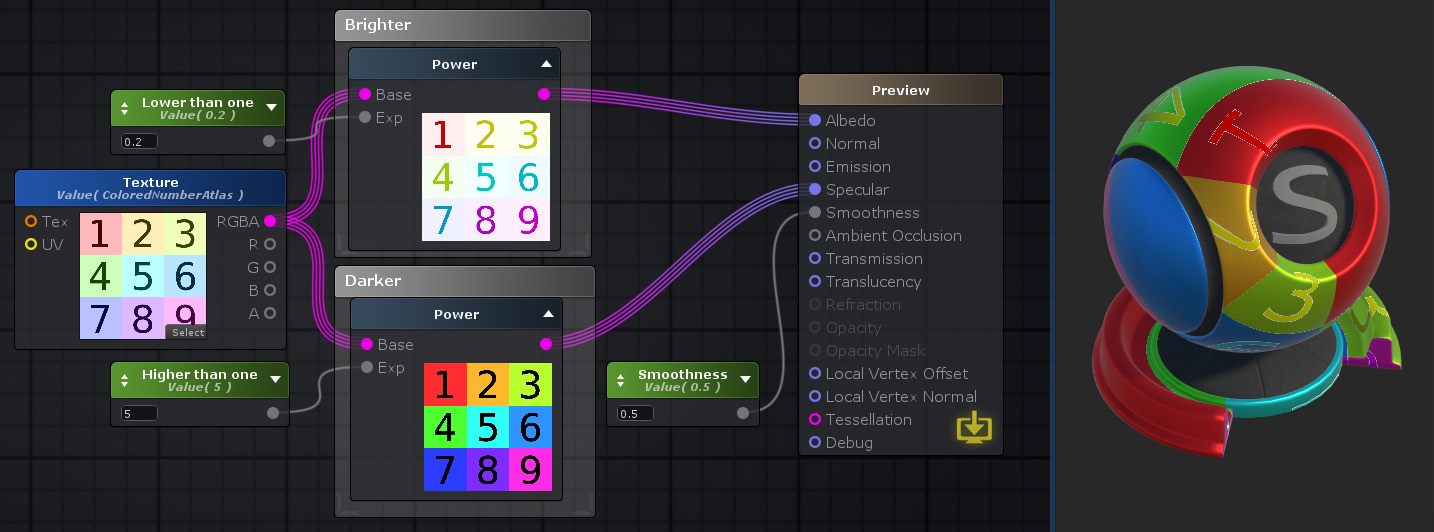## Power Node

The Power node ( shortcut: E key ) calculates a value using the exponentiation operator BaseExp.

• With Exp greater than 0 it corresponds to multiplying base by itself exp times, p.e Base = 2, Exp = 3 results in 2 x 2 x 2
• With Exp lower than 0 it corresponds to the inverse operation described above, p.e Base = 2, Exp = -3 results in 1/(2 x 2 x 2)
• On the other hand if Exp equals 0 then it will always output 1 independently of the Base value specified.

Power on multi-channel data types generates an output of the same type with the operator applied per-channel.

Note: Please check the following table for a complete behavior of the power operation depend on its input values.

Base Exp Result
< 0 any NaN
> 0 == 0 1
== 0 > 0 0
== 0 < 0 Infinite
> 0 < 0 1 / Base-Exp
== 0 == 0 Depends on GPU (0, 1 or NaN)Nodes used: Float, Texture Sample, Power

Node Parameter Description Default Value
Base Base value on the exponentiation operator. Only visible if the respective input port is not connected. 0
Exp Exponent value on the exponentiation operator. Only visible if the respective input port is not connected. 0
Safe Power When toggled on, assures that Base is always greater than 0 to avoid indeterminations/NaN. False

Input Port Description Type
Base Base value on the exponentiation operator. Float 
Exp Exponent value on the exponentiation operator. This port sets a minimum value of 0. Float

1. ^ Port automatically adapts to all connection types except Matrices and Sampler types.